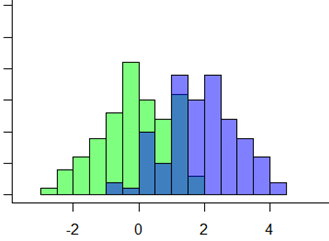# A Post-hoc Test for Kruskal-Wallis

If you’ve ever run a one-way analysis of variance (ANOVA), you’re familiar with post-hoc tests. The ANOVA omnibus test only tells you whether any groups differ in their means. But if you want to explore which specific group mean is different from which, you need to follow up with a post-hoc test.It’s not so simple though. Sometimes data don’t meet the distributional assumptions of ANOVA. What then? In this case, you might use a non-parametric analog to one-way ANOVA, the Kruskal-Wallis test.Kruskal-Wallis allows you to completely drop normality assumptions about the residual distribution. Rather than test whether the means of groups are equal, it tests whether the entire distribution of values are generally in the same location.

### How Kruskal-Wallis works

Kruskal-Wallis pools all Y values across all groups. Each Y value — sorted from smallest to largest — is substituted by a rank. Then ranks belonging to each group are averaged, to generate a mean rank.

The null hypothesis is that the difference between the mean ranks of all groups is 0. That is, all groups come from the same distribution of population data.

If you find that the groups are indeed different, you can test which specific group is different from which. You’ll need a post-hoc test for that.

For each post-hoc pairwise comparison test, you ask if the mean rank of one group is significantly different from the mean rank of another group. Arguably, the most popular rank test between two groups is the Mann-Whitney-Wilcoxon rank sum test. So is it a good idea to just run a Mann-Whitney-Wilcoxon rank sum test on each pair of groups?

As it turns out, it’s not ideal. Even if you apply a multiple comparison correction like Bonferroni.

Why? the Mann-Whitney-Wilcoxon test uses ranks of only two groups at a time. That’s different from the Kruskal-Wallis test statistic, which calculates ranks shared across all the groups.

So, in a very concrete way, using the Mann-Whitney-Wilcoxon as a post-hoc test would amount to using different data to test differences between any two groups.

### A Post-hoc test for Kruskal-Wallis: The Dunn’s Test

As it turns out there is a post-hoc tests that uses the same shared rankings as calculated by the Kruskal-Wallis, and it uses the same pooled variance that is implied by the null hypothesis of the Kruskal-Wallis test: the Dunn’s test. Thus, it uses the same data as the Kruskal-Wallis to test differences between any two groups.

Specifically, the Dunn’s (1964) z-test approximation is calculated as the difference in mean rank scores divided by the rank pooled variance estimate for two groups.

You can then apply multiple comparison adjustments to the Dunn’s test, like Bonferroni, Sidak, Holm, and Benjamini-Hochburg.

So the appropriate post-hoc test that should follow a Kruskal-Wallis test, is Dunn’s test, not Mann-Whitney-Wilcoxon.

by Ash RajeshThe Pathway: Steps for Staying Out of the Weeds in Any Data Analysis
Get the road map for your data analysis before you begin. Learn how to make any statistical modeling – ANOVA, Linear Regression, Poisson Regression, Multilevel Model – straightforward and more efficient.

1.jagdish says